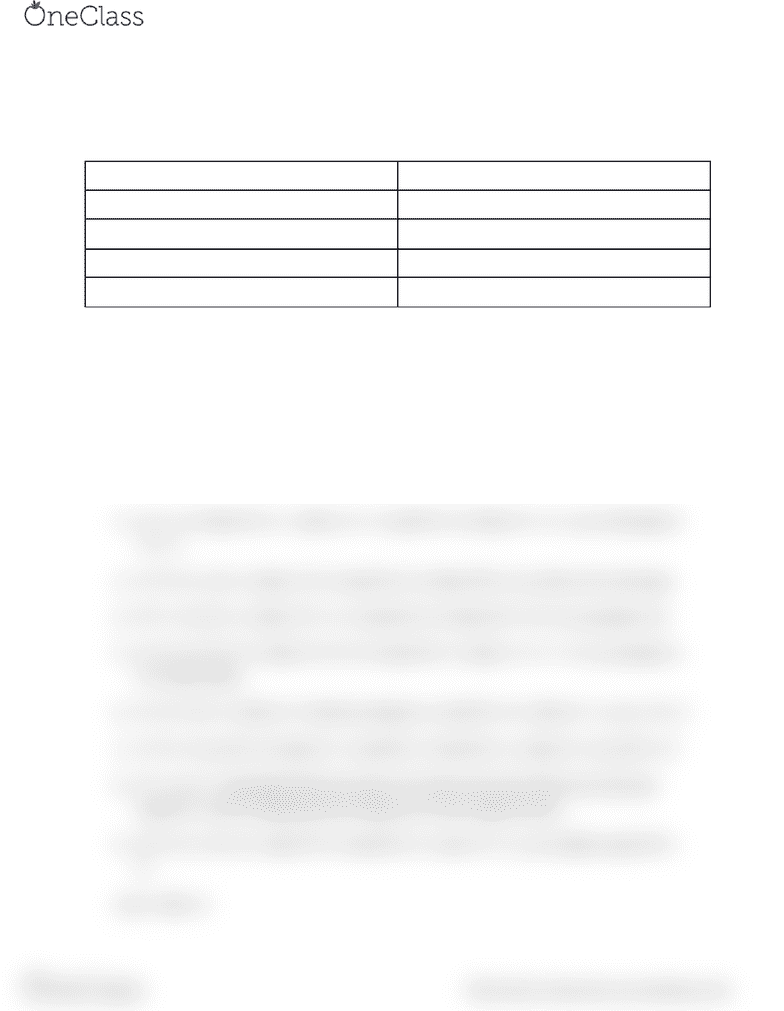# CPSC 259 Chapter 9.1: Rules for boolean algebra

54 views1 pagesRules for Boolean Algebra
There are 10 basic rules for Boolean Algebra
A + 0 = A A + 1 = 1
A * 0 = 0 A * 1 = A
A + A = A A * A = A
A + A’ = 1 A * A’ = 0
A’’ = A A + AB = A
These rules can be used to form further two rules:
1) A + A’B = A + B
2) (A+B)(A+C) = A*A + AC + AB + BC = A + BC
Proof for 1 to 10
1) A + 0 = A OR 0. Here if A is 1, then 1 + 0 = 0 and if A =0 , the 0 + 0 = 0, so in all
cases A + 0 = A.
2) A + 1 = A, Here if A = 1 , then 1+ 1 = 1 and if A = 0, then 0 + 1 = 1, so in all cases A
+1 = 1.
3) A * 0 = 0 , if A = 1 then 1* 0 = 0 and if A = 0, then 0*0 = 0, so this is 0 in all cases
4) A* 1 = A, if A = 1, then 1*1 = 1 = A and if A = 0, then 0*1 = 0 = A, so answer is A
5) A + A = A, if A = 0 , then 0 + 0 = 0 = A and if A = 1, then 1 + 1 = 1 = A, so answer is
A in both cases.
6) A + A’. If A = 1, then A’ = 0 and so answer is 1 and if A = 0 , then A’ = 1 ,so 1 + 0 = 1
7) A * A’ = 0, so if A = 0, then A’ = 1 and 0*1 = 0 and if A = 1, then A’ = 0, so 0*1 = 0
8) A’’ = A, so ‘’ essentially flips the value of A twice and since there are only two
options, 1 and 0, flipping twice brings you to the original value.
9) A* A = A , if A = 0 , then 0* 0 = 0 and if A = 1, the 1*1 = 1, so in either case A*A =
A.
10) A + AB = A
find more resources at oneclass.com
find more resources at oneclass.com
Unlock document

This preview shows half of the first page of the document.
Unlock all 1 pages and 3 million more documents.

Already have an account? Log in

# Get access

Grade+
\$10 USD/m
Billed \$120 USD annually
Homework Help
Class Notes
Textbook Notes
40 Verified Answers
Study Guides
1 Booster Class
Class+
\$8 USD/m
Billed \$96 USD annually
Homework Help
Class Notes
Textbook Notes
30 Verified Answers
Study Guides
1 Booster Class